# MCQs on Electric Circuits

##### Page 59 of 63. Go to page 1 2 3 4 5 6 7 8 9 10 11 12 13 14 15 16 17 18 19 20 21 22 23 24 25 26 27 28 29 30 31 32 33 34 35 36 37 38 39 40 41 42 43 44 45 46 47 48 49 50 51 52 53 54 55 56 57 58 59 60 61 62 63
01․ A battery of E Volts is supplying a steady current to a series circuit of total resistance RΩ and inductance L Henry. A part R1 of the total resistance is suddenly short circuited. Find the expression for current flowing through the battery subsequent to the operation.
i = {E / (R - R1)}[1-(R1 / R) *e- {(R - R1 )t/L}
i = {E / R1 - R)}(1- e - (R1 - R) t / L)
i = {E / R}[1-(R1 / R) *e - {(R - R1 )*t/L}
none of the above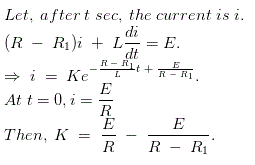02․ In above question, determine the current if E = 100 V, R = 20Ω, R1 = 10 Ω and L = 2 H at 0.5 sec after short circuit.
6.98.
- 6.28.
9.59.
- 9.59.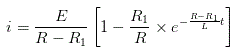03․ Find the total power absorbed by the resistor in the given circuit.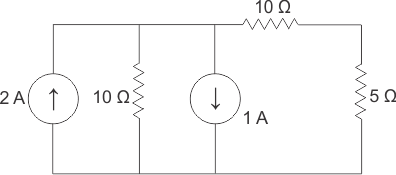6 W.
12 W.
24 W.
30 W.

Equivalent circuit can be drawn,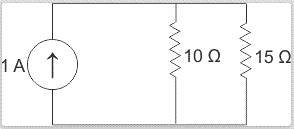P = I2R.

04․ In the circuit shown below if I = 2 A then find V?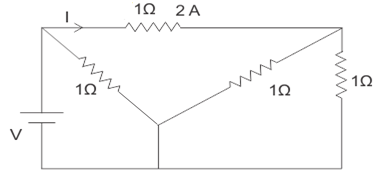5 V.
3 V.
2 V.
1 V.

The circuit can be redrawn as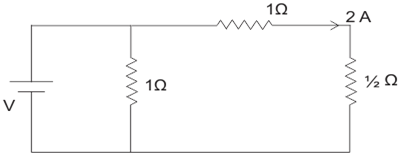05․ What is I1 in the given circuit?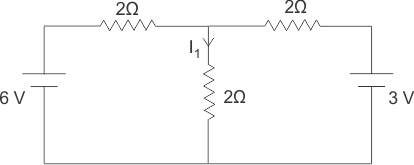0.5 A.
1 A.
1.5 A.
3 A.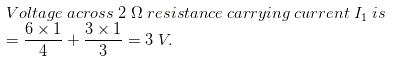06․ What is Va in the below circuit?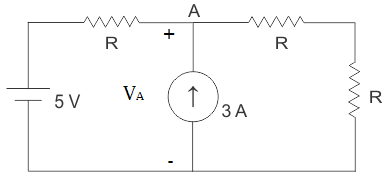(6R + 10) / 3.
(3R + 10) / 3.
(4R + 10) / 3.
(6R - 10) / 3.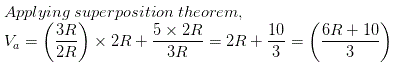07․ If L and C both are doubled than damping in above circuit will be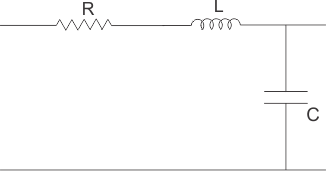doubled as compared to previous one.
halved as compared to previous one.
remains same.
none of the above.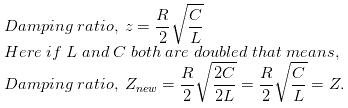08․ A capacitor is charged by a constant 10 mA current sources which is turned on for 1 second. Assuming the capacitor is initially charge free; determine the charge delivered to and the power supplied by the source if the capacitor has a value of 1 mF.
0.01C, 10MW.
0.01C and 5W.
0.1C, 10MW.
0.1C, 100MW.

Q = I×t; power = 0.5Q2t/C

09․ If the practical units of voltage and current wave each made 20 times as large as they are at present, what would be the consequent alternation in the size of the unit of capacitance?
20 times.
60 times.
200 times.
Nil.

Capacitance (C) = Q/V = It/V. Since I and V units are both charged by the same factor and time unit is not changed, hence capacitance unit is not affected.

10․ The speed of a DC motor is
directly proportional to flux and inversely proportional to back emf.
directly proportional to both back emf and flux.
directly proportional to back emf and flux.
directly proportional to back emf and inversely proportional flux.

Speed of DC motor is given by N = K X (Eb/φ). Where N = Speed in rpm, Eb = back emf and φ = flux per pole. In above relation, we get the speed of DC motor is directly proportional to its back emf and is inversely proportional to flux.

<<<5758596061>>>